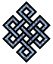#fail2ban bad ip database: ip 34.204.131.43

### | ip database | live view | stats | report | help | api key:

 ip: 34.204.131.43 hostname: ec2-34-204-131-43.compute-1.amazonaws.com country:[US] United States first reported: 16.11.2018 02:24.03 GMT+0200 last reported: 28.11.2018 14:19.52 GMT+0200 time period: 12d 11h 55m 49s total reports: 11 reported by: 3 host(s) filter(s): ssh (10) ssh (1) tor exit node no badips.com db Lookup## port scan of '34.204.131.43':

[-hide]
```# Nmap 6.40 scan initiated Fri Nov 16 02:25:02 2018 as: /usr/bin/nmap -sU -sS -O 34.204.131.43
Nmap scan report for ec2-34-204-131-43.compute-1.amazonaws.com (34.204.131.43)
Host is up (0.081s latency).
Not shown: 1000 open|filtered ports, 985 filtered ports
PORT     STATE  SERVICE
21/tcp   open   ftp
22/tcp   open   ssh
25/tcp   open   smtp
80/tcp   open   http
443/tcp  closed https
587/tcp  closed submission
1433/tcp closed ms-sql-s
3306/tcp closed mysql
3389/tcp open   ms-wbt-server
5500/tcp closed hotline
5510/tcp closed secureidprop
5900/tcp closed vnc
6000/tcp closed X11
8080/tcp closed http-proxy
8888/tcp closed sun-answerbook
No exact OS matches for host (If you know what OS is running on it, see http://nmap.org/submit/ ).
TCP/IP fingerprint:
OS:SCAN(V=6.40%E=4%D=11/16%OT=21%CT=443%CU=%PV=N%G=Y%TM=5BEE1C94%P=x86_64-p
OS:c-linux-gnu)SEQ(SP=104%GCD=1%ISR=10C%TI=Z%CI=I%TS=8)SEQ(SP=105%GCD=1%ISR
OS:=10C%TI=Z%TS=8)OPS(O1=M5B4ST11NW7%O2=M5B4ST11NW7%O3=M5B4NNT11NW7%O4=M5B4
OS:ST11NW7%O5=M5B4ST11NW7%O6=M5B4ST11)WIN(W1=68DF%W2=68DF%W3=68DF%W4=68DF%W
OS:5=68DF%W6=68DF)ECN(R=Y%DF=Y%TG=40%W=6903%O=M5B4NNSNW7%CC=Y%Q=)T1(R=Y%DF=
OS:Y%TG=40%S=O%A=S+%F=AS%RD=0%Q=)T2(R=N)T3(R=N)T4(R=Y%DF=Y%TG=40%W=0%S=A%A=
OS:Z%F=R%O=%RD=0%Q=)T5(R=Y%DF=Y%TG=40%W=0%S=Z%A=S+%F=AR%O=%RD=0%Q=)T6(R=Y%D
OS:F=Y%TG=40%W=0%S=A%A=Z%F=R%O=%RD=0%Q=)T7(R=Y%DF=Y%TG=40%W=0%S=Z%A=S+%F=AR
OS:%O=%RD=0%Q=)U1(R=N)IE(R=N)

OS detection performed. Please report any incorrect results at http://nmap.org/submit/ .
# Nmap done at Fri Nov 16 02:25:40 2018 -- 1 IP address (1 host up) scanned in 38.91 seconds
```
```Σ = 40 | Δt = 0.003870964050293s
```